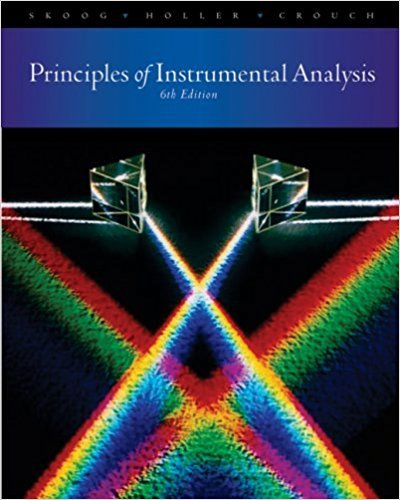×
×

# The accompanying absorption data were recorded at 390 nm in 1.00-cm cells foraISBN: 9780495012016 317

## Solution for problem 14-18 Chapter 14

Principles of Instrumental Analysis | 6th Edition

• Textbook Solutions
• 2901 Step-by-step solutions solved by professors and subject experts
• Get 24/7 help from StudySoup virtual teaching assistantsPrinciples of Instrumental Analysis | 6th Edition

4 5 1 333 Reviews
24
0
Problem 14-18

The accompanying absorption data were recorded at 390 nm in 1.00-cm cells fora continuous-variation study of the colored product formed between Cd'+ andthe eomplexing reagent R.(a) Find the ligand-to-metal ratio in the prodUCt.(b) Calculate an average value for the molar absorptivity of the complex andits uncertainty. Assume that in the linear portions of the plot the metal IScompletely complexed. ..... .(c) Calculate Kffor the complex using the stOlchlomctnc ratIO determmed m(a) and the absorption data at the point of intersectIon of the two extrapolatedlines.

Step-by-Step Solution:
Step 1 of 3

SPRING 2016 CHEM 112 MONTANO Chapter 1 Matter  Properties of matter are determined by the properties of the molecules and/or atoms it is composed of o Ex. Water (H O) Hydrogen Peroxide (H O ) 2 2 2  Water is stable, drinkable, and if you boil it, it will just evaporate  Hydrogen...

Step 2 of 3

Step 3 of 3

##### ISBN: 9780495012016

Since the solution to 14-18 from 14 chapter was answered, more than 219 students have viewed the full step-by-step answer. This full solution covers the following key subjects: . This expansive textbook survival guide covers 34 chapters, and 619 solutions. Principles of Instrumental Analysis was written by and is associated to the ISBN: 9780495012016. The full step-by-step solution to problem: 14-18 from chapter: 14 was answered by , our top Chemistry solution expert on 03/02/18, 06:21PM. The answer to “The accompanying absorption data were recorded at 390 nm in 1.00-cm cells fora continuous-variation study of the colored product formed between Cd'+ andthe eomplexing reagent R.(a) Find the ligand-to-metal ratio in the prodUCt.(b) Calculate an average value for the molar absorptivity of the complex andits uncertainty. Assume that in the linear portions of the plot the metal IScompletely complexed. ..... .(c) Calculate Kffor the complex using the stOlchlomctnc ratIO determmed m(a) and the absorption data at the point of intersectIon of the two extrapolatedlines.” is broken down into a number of easy to follow steps, and 84 words. This textbook survival guide was created for the textbook: Principles of Instrumental Analysis , edition: 6.

Unlock Textbook Solution